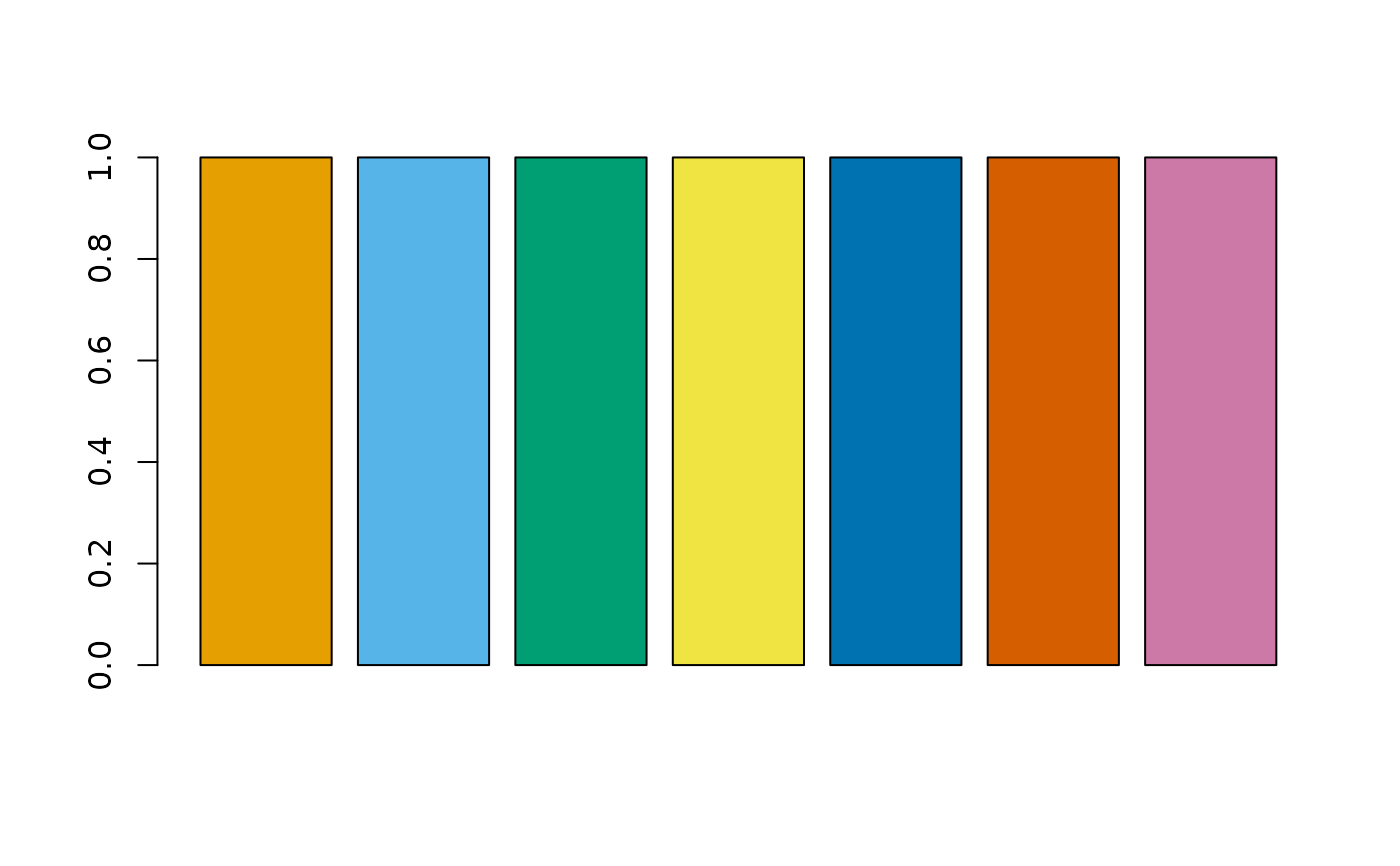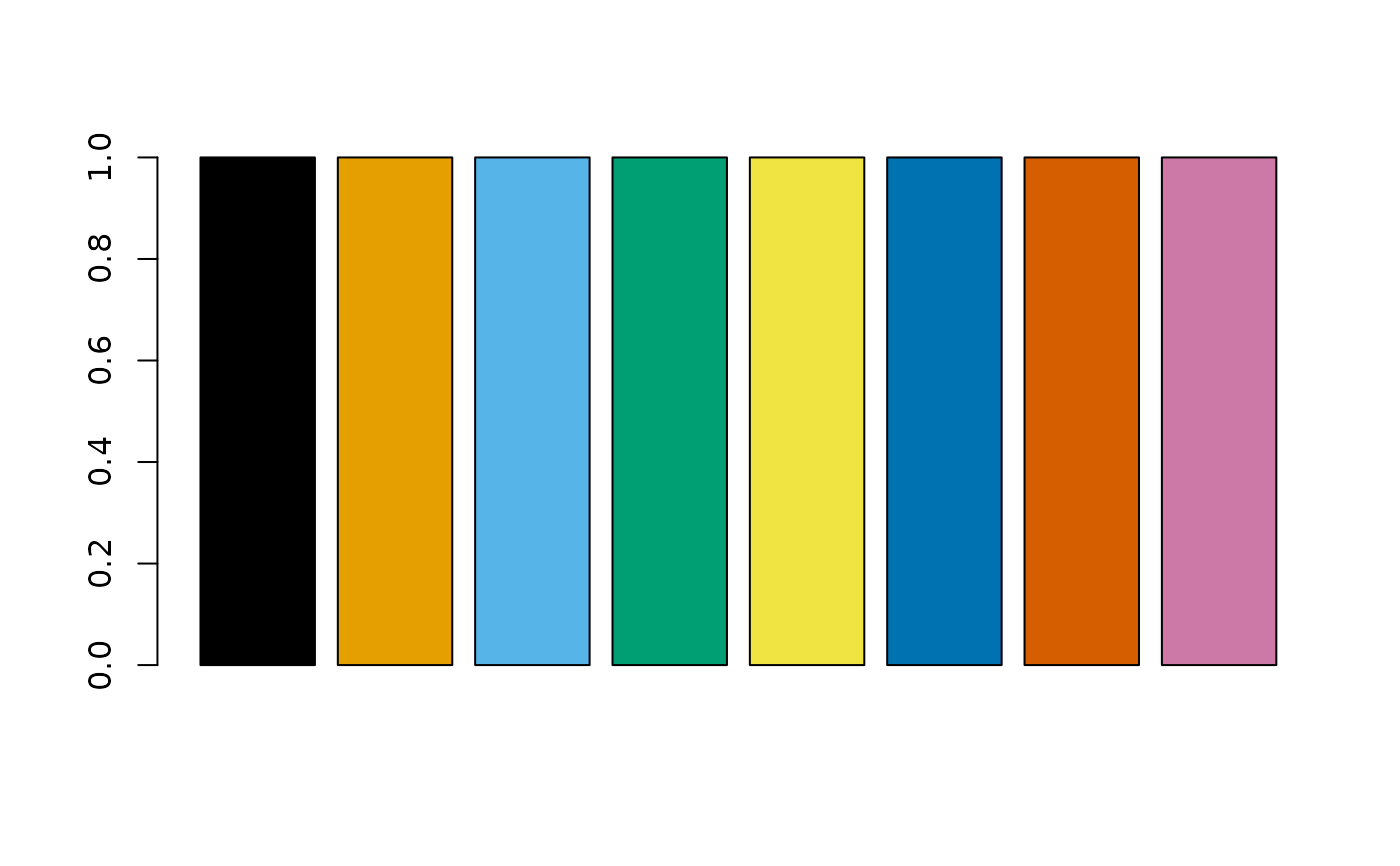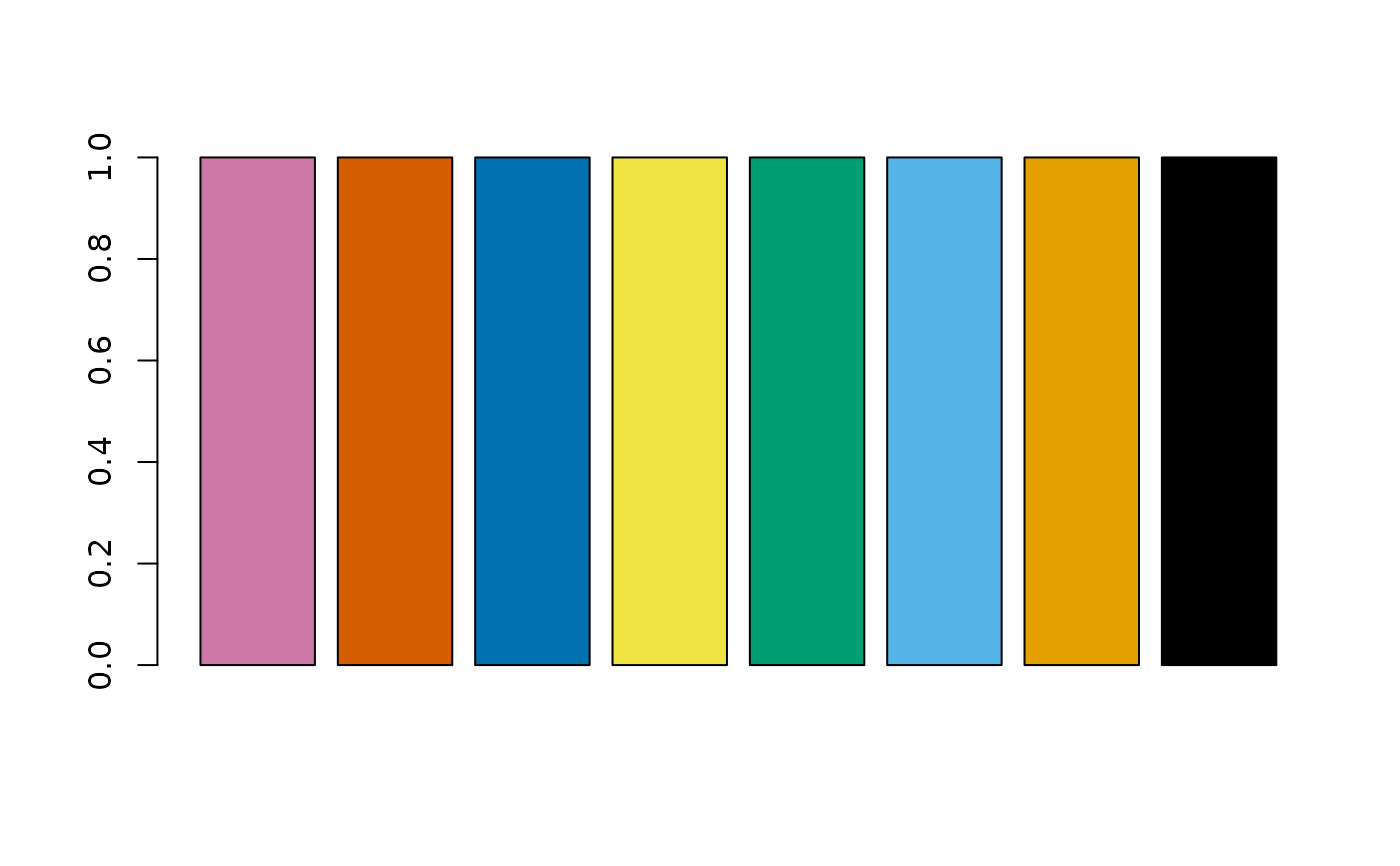Masataka Okabe and Kei Ito's Color Universal Design palette

cud(x, shift = TRUE, reverse = FALSE)

## Arguments

x vector, color index start from the second color in the CUD palette? reverse the order?

## Value

a vector of color hex values

## Examples

barplot(rep(1, 7), col = cud(1:7))barplot(rep(1, 8), col = cud(1:8, shift = FALSE))barplot(rep(1, 8), col = cud(1:8, shift = FALSE, reverse = TRUE))